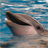# QlikView App Dev

Discussion Board for collaboration related to QlikView App Development.

Announcements
QlikWorld 2023, a live, in-person thrill ride. Save \$300 before February 6: REGISTER NOW!
cancel
Showing results for
Did you mean:Contributor III

## Pivot table issue

Hi ,

I created a pivot table with four dimensions and 2 Kpi's.

i can see the correct values, when i expand  all the dimensions at one time.

if i expand dimension by dimension wise, i am not able to see the correct kpi values.

can any one help me.

22 RepliesChampion III

Will have to look into it. Will get back in a while. However mentioning Sunny if he has any quick resolution for this, i vaguely remember he did fix something like this long ago.

Hey stalwar1‌ would you mind looking into this please.MVP

May be this?

=if(((count(total aggr(  sum(TotalPaid),ServDate,MealPeriod,[Time Slot]))) - (If(Dimensionality() = 3, Aggr(Rank(Total  sum(TotalPaid)/v1), ServDate,MealPeriod,[Time Slot]), Rank(Total  sum(TotalPaid)/v1))))

/

(count(total aggr(  sum(TotalPaid),ServDate,MealPeriod,[Time Slot])))<0.1,'0-10%',

if(((count(total aggr(  sum(TotalPaid),ServDate,MealPeriod,[Time Slot]))) - (If(Dimensionality() = 3, Aggr(Rank(Total  sum(TotalPaid)/v1), ServDate,MealPeriod,[Time Slot]), Rank(Total  sum(TotalPaid)/v1))))

/

(count(total aggr(  sum(TotalPaid),ServDate,MealPeriod,[Time Slot])))<0.2,'10-20%',

if(((count(total aggr(  sum(TotalPaid),ServDate,MealPeriod,[Time Slot]))) - (If(Dimensionality() = 3, Aggr(Rank(Total  sum(TotalPaid)/v1), ServDate,MealPeriod,[Time Slot]), Rank(Total  sum(TotalPaid)/v1))))

/

(count(total aggr(  sum(TotalPaid),ServDate,MealPeriod,[Time Slot])))<0.3,'20-30%',

if(((count(total aggr(  sum(TotalPaid),ServDate,MealPeriod,[Time Slot]))) - (If(Dimensionality() = 3, Aggr(Rank(Total  sum(TotalPaid)/v1), ServDate,MealPeriod,[Time Slot]), Rank(Total  sum(TotalPaid)/v1))))

/

(count(total aggr(  sum(TotalPaid),ServDate,MealPeriod,[Time Slot])))<0.4,'30-40%',

if(((count(total aggr(  sum(TotalPaid),ServDate,MealPeriod,[Time Slot]))) - (If(Dimensionality() = 3, Aggr(Rank(Total  sum(TotalPaid)/v1), ServDate,MealPeriod,[Time Slot]), Rank(Total  sum(TotalPaid)/v1))))

/

(count(total aggr(  sum(TotalPaid),ServDate,MealPeriod,[Time Slot])))<0.5,'40-50%',

if(((count(total aggr(  sum(TotalPaid),ServDate,MealPeriod,[Time Slot]))) - (If(Dimensionality() = 3, Aggr(Rank(Total  sum(TotalPaid)/v1), ServDate,MealPeriod,[Time Slot]), Rank(Total  sum(TotalPaid)/v1))))

/

(count(total aggr(  sum(TotalPaid),ServDate,MealPeriod,[Time Slot])))<0.6,'50-60%',

if(((count(total aggr(  sum(TotalPaid),ServDate,MealPeriod,[Time Slot]))) - (If(Dimensionality() = 3, Aggr(Rank(Total  sum(TotalPaid)/v1), ServDate,MealPeriod,[Time Slot]), Rank(Total  sum(TotalPaid)/v1))))

/

(count(total aggr(  sum(TotalPaid),ServDate,MealPeriod,[Time Slot])))<0.7,'60-70%',

if(((count(total aggr(  sum(TotalPaid),ServDate,MealPeriod,[Time Slot]))) - (If(Dimensionality() = 3, Aggr(Rank(Total  sum(TotalPaid)/v1), ServDate,MealPeriod,[Time Slot]), Rank(Total  sum(TotalPaid)/v1))))

/

(count(total aggr(  sum(TotalPaid),ServDate,MealPeriod,[Time Slot])))<0.8,'70-80%',

if(((count(total aggr(  sum(TotalPaid),ServDate,MealPeriod,[Time Slot]))) - (If(Dimensionality() = 3, Aggr(Rank(Total  sum(TotalPaid)/v1), ServDate,MealPeriod,[Time Slot]), Rank(Total  sum(TotalPaid)/v1))))

/

(count(total aggr(  sum(TotalPaid),ServDate,MealPeriod,[Time Slot])))<0.9,'80-90%','90-100%')))))))))Champion III

Thanks Sunny. That is awesome.

Ravi, as mentioned before anyways you are getting your count totals from your variable v1. You can just use this in your spend % to make it more comprehensive. Just replaced that count total with variable v1.

=if(((v1) - (If(Dimensionality() = 3, Aggr(Rank(Total  sum(TotalPaid)/v1), ServDate,MealPeriod,[Time Slot]), Rank(Total  sum(TotalPaid)/v1))))/(v1)<0.1,'0-10%',

if(((v1) - (If(Dimensionality() = 3, Aggr(Rank(Total  sum(TotalPaid)/v1), ServDate,MealPeriod,[Time Slot]), Rank(Total  sum(TotalPaid)/v1)))) /(v1)<0.2,'10-20%',

if(((v1) - (If(Dimensionality() = 3, Aggr(Rank(Total  sum(TotalPaid)/v1), ServDate,MealPeriod,[Time Slot]), Rank(Total  sum(TotalPaid)/v1)))) /(v1)<0.3,'20-30%',

if(((v1) - (If(Dimensionality() = 3, Aggr(Rank(Total  sum(TotalPaid)/v1), ServDate,MealPeriod,[Time Slot]), Rank(Total  sum(TotalPaid)/v1)))) /(v1)<0.4,'30-40%',

if(((v1) - (If(Dimensionality() = 3, Aggr(Rank(Total  sum(TotalPaid)/v1), ServDate,MealPeriod,[Time Slot]), Rank(Total  sum(TotalPaid)/v1)))) /(v1)<0.5,'40-50%',

if(((v1) - (If(Dimensionality() = 3, Aggr(Rank(Total  sum(TotalPaid)/v1), ServDate,MealPeriod,[Time Slot]), Rank(Total  sum(TotalPaid)/v1)))) /(v1)<0.6,'50-60%',

if(((v1) - (If(Dimensionality() = 3, Aggr(Rank(Total  sum(TotalPaid)/v1), ServDate,MealPeriod,[Time Slot]), Rank(Total  sum(TotalPaid)/v1)))) /(v1)<0.7,'60-70%',

if(((v1) - (If(Dimensionality() = 3, Aggr(Rank(Total  sum(TotalPaid)/v1), ServDate,MealPeriod,[Time Slot]), Rank(Total  sum(TotalPaid)/v1)))) /(v1)<0.8,'70-80%',

if(((v1) - (If(Dimensionality() = 3, Aggr(Rank(Total  sum(TotalPaid)/v1), ServDate,MealPeriod,[Time Slot]), Rank(Total  sum(TotalPaid)/v1)))) /(v1)<0.9,'80-90%','90-100%')))))))))Contributor III
Author

Thanks a lot Sunny and Viswanath.

Its working fine.MVP

Qlik Community Tip: Marking Replies as Correct or Helpful

Best,

SunnyMVP

And his name is VishwarathContributor III
Author

Hi Sunny,

By Default the first dimension is showing 90-100%, is it possible to restrict this?it suppose to show calendar date wise.While expanding , it is working fine with other dimensions.MVP

Not sure I understand your question? What do you want to see instead of 90-100%?Champion III

Your spend is calculated based on the IF condition for Total spend and value your variable holds with a range.Contributor III
Author

Every calendar date has its own %Spend Value, but here it is same for all the dates.Community Browser